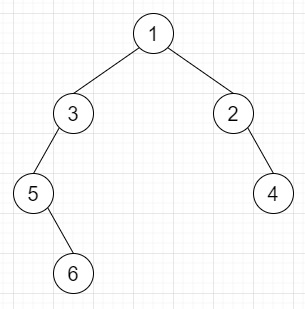# Encode N-ary Tree to Binary Tree in C++

Suppose we have a N-ary tree. We have to encode that tree into one binary. We also have to make deserializer to deserialize the binary tree to N-ary tree.

So, if the input is likethen the output will beTo solve this, we will follow these steps −

• Define a function encode(), this will take root,

• if root is valid, then −

• return null

• node = new tree node with value of root

• if size of children of root is not 0, then −

• left of node := encode(children of root)

• curr = left of node

• for initialize i := 1, when i < size of children of root, update (increase i by 1), do −

• right of node := encode(children[i] of root)

• curr := right of curr

• return node

• Define a function decode(), this will take root,

• if root is not present, then −

• return NULL

• node := new node with val of root

• curr := left of root

• while curr is non-zero, do −

• insert decode(curr) at the end of children of node

• curr := right of curr

• return node

## Example

Let us see the following implementation to get a better understanding −

Live Demo

#include <bits/stdc++.h>
using namespace std;
class TreeNode {
public:
int val;
TreeNode *left, *right;
TreeNode(int data) {
val = data;
left = NULL;
right = NULL;
}
};
void inord(TreeNode *root) {
if (root != NULL) {
inord(root->left);
cout << root->val << " ";
inord(root->right);
}
}
class Node {
public:
int val;
vector<Node*> children;
Node() {}
Node(int _val) {
val = _val;
}
Node(int _val, vector<Node*> _children) {
val = _val;
children = _children;
}
};
string n_ary_to_str(Node *root){
string ret = "";
if(root){
ret = ret + to_string(root->val);
if(root->children.size() > 0){
ret += "[";
for(Node* child : root->children){
ret += n_ary_to_str(child) + ", ";
}
ret += "]";
}
}
return ret;
}
class Codec {
public:
TreeNode* encode(Node* root) {
if(!root) return NULL;
TreeNode* node = new TreeNode(root->val);
if(root->children.size()){
node->left = encode(root->children);
}
TreeNode* curr = node->left;
for(int i = 1; i < root->children.size(); i++){
curr->right = encode(root->children[i]);
curr = curr->right;
}
return node;
}
Node* decode(TreeNode* root) {
if(!root) return NULL;
Node* node = new Node(root->val);
TreeNode* curr = root->left;
while(curr){
node->children.push_back(decode(curr));
curr = curr->right;
}
return node;
}
};
main() {
Codec ob;
Node n5(5), n6(6);
Node n3(3); n3.children.push_back(&n5); n3.children.push_back(&n6);
Node n2(2), n4(4);
Node n1(1); n1.children.push_back(&n3); n1.children.push_back(&n2);
n1.children.push_back(&n4);
cout << "Given Tree: " << n_ary_to_str(&n1) << endl;
cout << "Serialized Binary Tree: ";
TreeNode *root = ob.encode(&n1);
inord(root);
cout << endl;
Node *deser = ob.decode(root);
cout << "Deserialized Tree: " << n_ary_to_str(deser);
}

## Input

Node n5(5), n6(6);
Node n3(3); n3.children.push_back(&n5); n3.children.push_back(&n6);
Node n2(2), n4(4);
Node n1(1); n1.children.push_back(&n3); n1.children.push_back(&n2);
n1.children.push_back(&n4);

## Output

Given Tree: 1[3[5, 6, ], 2, 4, ]
Serialized Binary Tree: 5 6 3 2 4 1
Deserialized Tree: 1[3[5, 6, ], 2, 4, ]